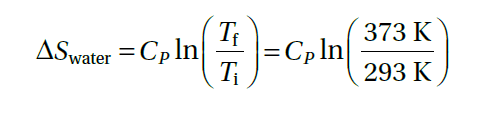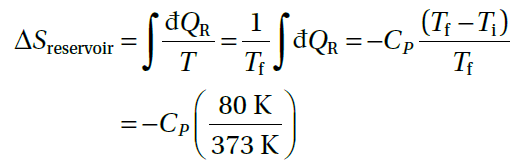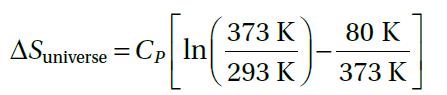# Is this entropy problem right? (heating a beaker of water)

LCSphysicist
Homework Statement:
Consider heating a beaker of water, with heat capacity CP, from Ti = 293 K to Tf = 373 K, , bringing up a whole series of reservoirs between these temperature.
Relevant Equations:
\nI am a little confused why does the temperature was took out of the integration, is there an error in the solution?

Mentor
Homework Statement:: Consider heating a beaker of water, with heat capacity CP, from Ti = 293 K to Tf = 373 K, , bringing up a whole series of reservoirs between these temperature.
Relevant Equations:: \n

View attachment 272678
View attachment 272679
View attachment 272680
I am a little confused why does the temperature was took out of the integration, is there an error in the solution?
An ideal constant temperature reservoir is defined as one of infinite heat capacity and infinite thermal conductivity (so that its temperature is everywhere uniform). If you used the equation for the water and applied it to the reservoir, taking the limit of infinite heat capacity for the reservoir, you would arrive at the same equation as the one you have written for the reservoir.

•LCSphysicist
Homework Helper
Gold Member
2022 Award
Hi. I agree the working shown is not clear. Sorry if my answer is a bit long/clumsy but see if it helps...

##dS ≥ \frac {dQ}{T}## The ‘equals’ applies to a reversible heat transfer and the ‘greater than’ applies to an irreversible heat transfer..

For a reversible transfer, the temperature difference must be infinitesimally small.

In this question you are (by implication) being asked for the minimum possible entropy change, so you must consider only reversible transfers. The question should have stated 'minimum'.

Suppose you have a single reservoir at temperature ##T_f## and remove an amount of heat, ##Q##, from it. The reservoir's entropy change is ##\frac {-Q}{T_f}##.

In this question we can’t use a single reservoir because we need an infinitesimal temperature difference between reservoir and beaker in order to have a reversible heat transfer. So instead of a single reservoir at ##T_f##, we make the heating process reversible - we use an infinite series of reservoirs starting with a reservoir at temperature ##T_i + dT## and finishing with a reservoir at temperature ##T_f##.

This is what is meant by ##\int \frac {dQ_r}{T}##

Doing it this way, the integral is the same as the entropy change of the single reservoir above. It is ##\frac{-Q}{T_f}##. But don't ask me to prove it!

The heat gained by the water is ##Q = C_p(T_f – T_i)## so this is the heat lost by the series of reservoirs.

##ΔS_{reservoir} =-Q/T_f = -\frac{C_p(T_f – T_i)}{T_f}##

•LCSphysicist
Mentor
Hi. I agree the working shown is not clear. Sorry if my answer is a bit long/clumsy but see if it helps...

##dS ≥ \frac {dQ}{T}## The ‘equals’ applies to a reversible heat transfer and the ‘greater than’ applies to an irreversible heat transfer..

For a reversible transfer, the temperature difference must be infinitesimally small.

In this question you are (by implication) being asked for the minimum possible entropy change, so you must consider only reversible transfers. The question should have stated 'minimum'.

Suppose you have a single reservoir at temperature ##T_f## and remove an amount of heat, ##Q##, from it. The reservoir's entropy change is ##\frac {-Q}{T_f}##.

In this question we can’t use a single reservoir because we need an infinitesimal temperature difference between reservoir and beaker in order to have a reversible heat transfer. So instead of a single reservoir at ##T_f##, we make the heating process reversible - we use an infinite series of reservoirs starting with a reservoir at temperature ##T_i + dT## and finishing with a reservoir at temperature ##T_f
This is what is meant by ##\int \frac {dQ_r}{T}##

Doing it this way, the integral is the same as the entropy change of the single reservoir above. It is ##\frac{-Q}{T_f}##. But don't ask me to prove it!

The heat gained by the water is ##Q = C_p(T_f – T_i)## so this is the heat lost by the series of reservoirs.

##ΔS_{reservoir} =-Q/T_f = -\frac{C_p(T_f – T_i)}{T_f}##
This answer makes some sense, but not for the entropy change of the series of reservoirs. If the heat transfer is reversible as you describe it, then the entropy change of the series of reservoirs is minus the entropy change of the water. But, if only a single reservoir is used at the final temperature (so that the process is irreversible), then the OPs equations correctly apply to both the water and the single reservoir.

•LCSphysicist
Homework Helper
Gold Member
2022 Award
.
If the heat transfer is reversible as you describe it, then the entropy change of the series of reservoirs is minus the entropy change of the water. But, if only a single reservoir is used at the final temperature (so that the process is irreversible), then the OPs equations correctly apply to both the water and the single reservoir.

Agreed. If my approach were correct there would be zero net ('universe) entropy change, which is wrong.

The original question mentions "a whole series of reservoirs" so I don’t know how to justify using only the entropy change of a single reservoir at ##T_f##.

With this in mind, I was hoping to explain why ##\int \frac {dQ_r}{T}## gets turned into ##\frac{1}{T_f} \int {dQ_r}##. But I can't.

Interesting that the 'd's (differentials) in the original text have strike-throughs - don't know the significance (if any) of this symbol.

•LCSphysicist
Mentor
Agreed. If my approach were correct there would be zero net ('universe) entropy change, which is wrong.

The original question mentions "a whole series of reservoirs" so I don’t know how to justify using only the entropy change of a single reservoir at ##T_f##.

With this in mind, I was hoping to explain why ##\int \frac {dQ_r}{T}## gets turned into ##\frac{1}{T_f} \int {dQ_r}##. But I can't.

Interesting that the 'd's (differentials) in the original text have strike-throughs - don't know the significance (if any) of this symbol.
My guess is that the OP was asked to determine the entropy change of the system, the surroundings, and the universe for the irreversible process in which the water is put in contact with an ideal constant temperature reservoir at 373 K, and allowed to equilibrate.

To get the entropy change for the water, he had to devise an alternate reversible process between the same two end states, and this involved substituting for the original reservoir the sequence of constant temperature reservoirs at slightly different temperatures.

Since the original reservoir was ideal, its entropy change for the irreversible process was just equal to the original heat transfer divided by its constant temperature. In other words, all the irreversibility for the original process occurred within the water.

•LCSphysicist and Steve4Physics
LCSphysicist
The solution was provided by the book i was using. I post it because i disagree with the solution. Is not an ideal reservoir in fact, it is just an reservoir. Assuming the entropy is a function of state, to calc the entropy was used a series of reservoirs with Temperature T+dt, making the process reversible and able to calc the difference of entropy.
The problem is this Tf, as you can see, and as @Chestermiller pointed, when he assumed the reservoir was at 373 K, the answer makes sense only in this way. But this is not the case, so the confusion with the answer.
Agreed. If my approach were correct there would be zero net ('universe) entropy change, which is wrong.

The original question mentions "a whole series of reservoirs" so I don’t know how to justify using only the entropy change of a single reservoir at ##T_f##.

With this in mind, I was hoping to explain why ##\int \frac {dQ_r}{T}## gets turned into ##\frac{1}{T_f} \int {dQ_r}##. But I can't.

Interesting that the 'd's (differentials) in the original text have strike-throughs - don't know the significance (if any) of this symbol.
The strike means inexact differential, you can't find an equation like Q = f(T) [Q = mcdT is function of dT], that is, an function representing the heat in function of other variable. Because it does not makes sense :)

Mentor
When they say reservoir, they mean ideal reservoir as I described it.

•LCSphysicist
Mentor
Can you please provide the exact wording of the complete problem? Then we can determine whether the answer they gas is correct or not.

•LCSphysicist
LCSphysicist
Can you please provide the exact wording of the complete problem? Then we can determine whether the answer they gas is correct or not.
Oh yes, apparently they are assuming a reservoir with 100°C but calculating the entropy as bringing up a whole series of reservoirs between the temperature of the reservoir and the water. As you right pointed (ignore the post above, i was about to sleep :v). The problem was that i forgot this detail and was stuck in the calculation of the entropy, but now it makes more sense. Thank you